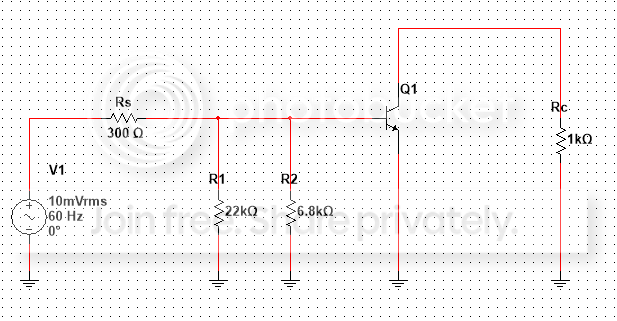# Transistor Amplifier Circuit

I need your help

Given:

• AC beta (Bac) = 160
• Ie = 3.8 mA

Find :

• a) Rin (TOTAL)

b) Rin (BASE)

c) Av (voltage gain)I am uncertain of the process.

I have Rin (Base) = Rs + R1||R2 or is it the Rin (total)? If not, where is Rin (total)?

Then I compute for Ib = Vs/Rin(Base)

Substitute Bac = Ic/Ib values to compute for Ic

Then formula for re'=25mV/Ie

Then c) Av = Rc/re'

• You speak of Ib and such, but we see a mosfet here. Plus the biasing circuit is missing, and beta is not enough to solve the rest of the circuit. Fix your question please. – Vladimir Cravero May 3 '15 at 14:09
• sorry i will revise it I didn't notice the missing given – Brian Sanchez May 3 '15 at 14:12
• Because I feel you are a beginner (basic errors) can you show the proper circuit digram including power supply and the proper connections of R1 (or R2) to that supply. Showing an equivalent ac circuit does not convince me of your interpretation. – Andy aka May 3 '15 at 14:26
• Yes I am. The circuit was given as homework for my electronics class. I honestly don't know what proper circuit diagram will look like from the given circuit. :( – Brian Sanchez May 3 '15 at 14:38
• Replace that lonely lost MOSFET with the small signal BJT model you learnt in class- one with a dependent source. – Spehro Pefhany May 3 '15 at 14:43

## 1 Answer

In order to compute the given quantities, You must be aware of small signal equivalent circuit of various BJT configurations. A little more experienced users can directly determine these quantities from the given circuit diagram itself.

Here is a commonly used circuit :It is called as Voltage Divider bias and has an equivalent small signal ofHow do you get this small signal ckt ?


First replace all the Dc supplies by Gnd. They have no role to play in ac analysis except for biasing the BJT in proper region.

Next see that R1 and R2 are both connected with one end tied to base and other end to Gnd. So they appear in parallel.

The small signal resistance called re is connected to the emitter. Its value is Ie / Vt . However, it is 'reflected' to the input side by a factor of (B + 1). The Emitter resistance RE is also reflected similarly. However, here it is bypassed by the capacitor which acts as a short circuit for small ac signals, and hence does not appear in the picture.

The resistance ro is there to model the Early Effect and has large value ( in 10's of K ohm)

Finding various Quantities


As a rule of thumb, it is always best to begin by finding the Emitter current, which depends on your particular biasing scheme. Here however, you are already given its value as 3.8 mA.

Next determine the value of re as Ie / Vt and optionally, transconductance gm, which here equals (1 / re ).

So the base resistance is parallel combo of R1 and R2. Call it Zb (or anything).

The input resistance is ( Zb || B.re ) + Source Resistance Rs.

The Output resistance is ro || Rc . Call it Zo

The voltage gain is (gm.Zo)